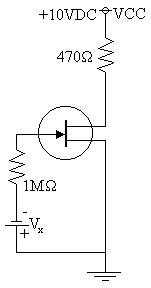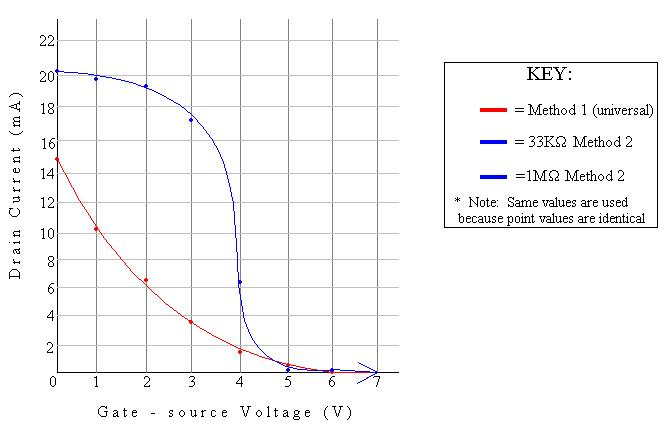EL - 200

Electronic Devices

Lab #5: JFET Circuits

Andrew Buettner

March 6, 2003Objective

The objective of this lab is to construct a fixed bias circuit using a JFET. Then to use that circuit to determine if a change in the resistor connected to the G connection will affect the operation of the circuit. Then to plot the current flowing through the transistor as a function of voltage.Equipment Used

1) Trainer #1278

2) Multimeter #1261

3) 2N4092 N - channel JFET

4) 470W resistor

5) 33KW resistor

6) 1MW resistorProcedure

1) Assemble the following circuit:2) Set VX to be 0V and Measure VDS

3) Calculate ID, IS, and IG using the following values:

a) IDSS = 15mA

b) VP = -6V

4) Repeat steps 2 and 3 using the following voltages:

a) -1V

b) -2V

c) -3V

d) -4V

e) -5V

f) -6V

5) Repeat steps 2 through 4 using a 33KW resistor in place of the 1MW resistorData

1) Table 1: Values for 1MW resistor:

VX

VDS

ID

IS

IG

0V

.517V

15mA

15mA

0mA

-1V

.639V

10.4mA

10.4mA

0mA

-2V

.877V

6.67mA

6.67mA

0mA

-3V

1.936V

3.75mA

3.75mA

0mA

-4V

7.08V

1.67mA

1.67mA

0mA

-5V

9.96V

.417mA

.417mA

0mA

-6V

9.97V

0mA

0mA

0mA

2) Table 2: Values for 33KW resistor:

VX

VDS

ID

IS

IG

0V

.477V

15mA

15mA

0mA

-1V

.636V

10.4mA

10.4mA

0mA

-2V

.869V

6.67mA

6.67mA

0mA

-3V

1.935V

3.75mA

3.75mA

0mA

-4V

7.07V

1.67mA

1.67mA

0mA

-5V

9.96V

.417mA

.417mA

0mA

-6V

9.97V

0mA

0mA

0mA

3) Table 3: Comparison of ID through different methods of calculation: * See Conclusions *

R = 1MW R = 33KW

VX

Method 1

Method 2

Method 1

Method 2

0

15mA

20.2mA

15mA

20.3mA

1

10.4mA

19.9mA

10.4mA

19.9mA

2

6.67mA

19.4mA

67mA

19.4mA

3

3.75mA

17.2mA

3.75mA

17.2mA

4

1.67mA

6.21mA

1.67mA

6.21mA

5

.417mA

.0851mA

.417mA

.0851mA

6

0mA

.0638mA

0mA

.0638mA

3) Diagram 1: Schematic 1, enlarged view:4) Diagram 2: Graph of VGS vs. IDAnswers to Lab Questions

1) Q: Does changing the value of RG have a significant effect on the operation of the circuit and why?

A: No, The Drain - Source voltages are extremely similar between the two data tables, this is expected as IG is always taken to be 0mA causing the resistor to an insignificant component.

2) Q: Is the assumption that VP = -6V a valid one?

A: No, the tables show that the pinch off voltage occurs between VGS = -4V and VGS = -5V.Conclusions

From this lab it is concluded that the value of the resistor attached to the gate input of a JFET has no effect on the operation of the JFET. I would like to comment that I took the opportunity to calculate ID using two different methods: The first was the expected one using Shockley's equation. The second method was to calculate the voltage drop across the drain resistor and, using that value, calculate the resulting current. I was surprised at the difference in results, however i do believe that the results given from the second method are more applicable and yield a more accurate curve. The curve yielded is also closer to the actual curve expected from a JFET transistor.Attachments

1) Original handout

2) Original lab data

3) Calculations